# Linearity assessment in multivariate analysis

Modified on Mon, 23 Nov 2020 at 10:47 AM

Linear regression implies that there is a linear relationship (linearity) between the variable to explain and the numeric explanatory variables. Logistic regression implies that there is a linear relationship (called log-linearity) between the log-odds of the variables to explain and the numeric explanatory variables.

If these conditions aren't respected, the coefficients computed could be uncertain, that is why it is really important to assess the linearity of a linear model or the log-linearity of a logistic model before any interpretation of the results.

# Is the red line generally within the green zone?

## Linear regression

Taking for example the following model: one wants to explain the variable to explain "Numbers of asthma attacks in the last 12 months" by the explanatory variables "Age", "Sex" (female/male), "Pack year", "Disease-modifying treatments" (yes/no), "Years since diagnosis", "Number of asthma diagnosis in family", "Therapeutic education sessions in the past 6 months" and "Alcohol per day (g/day)".

YES, it is clearly within the green zone

Even if the red line is out of the green zone some times, the  relationship is generally linear.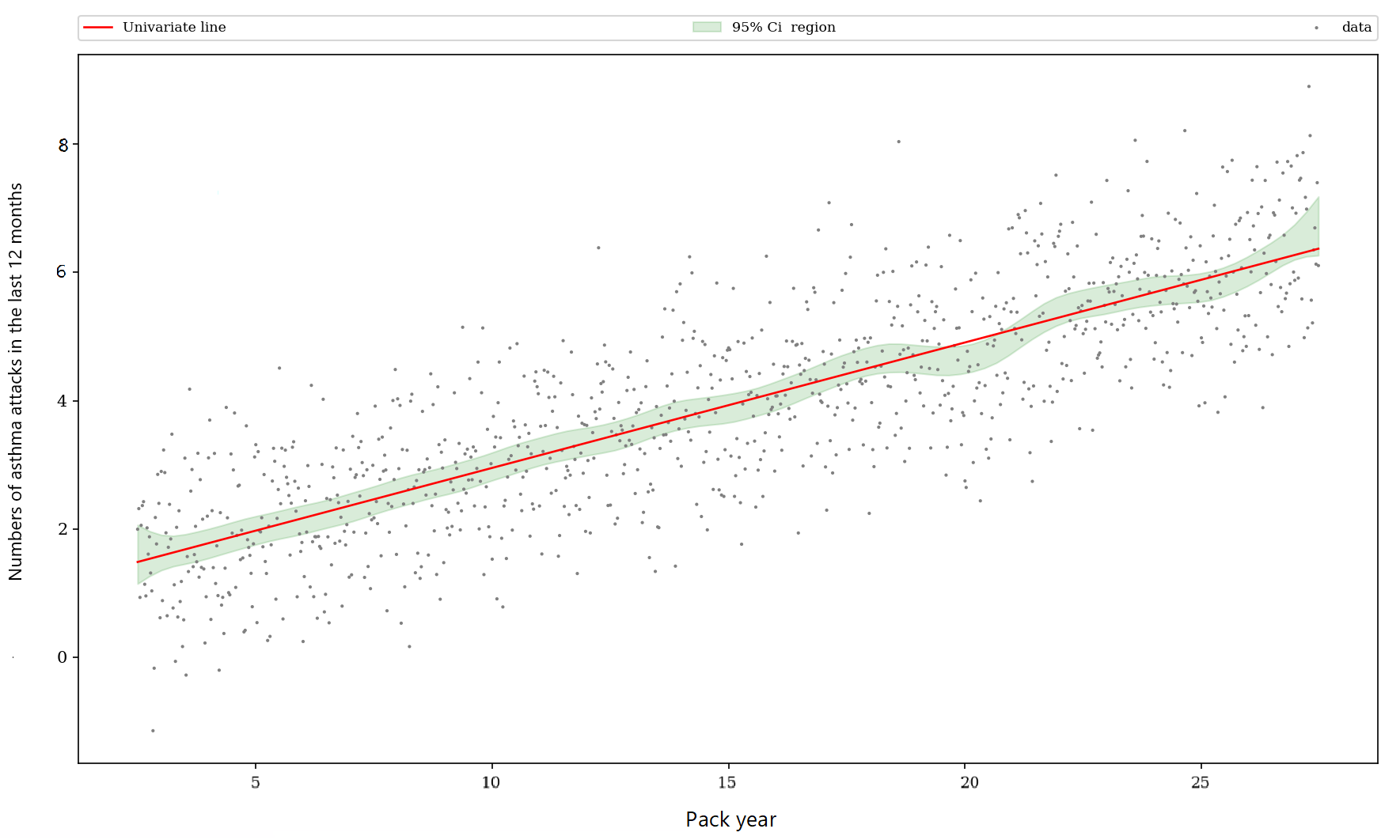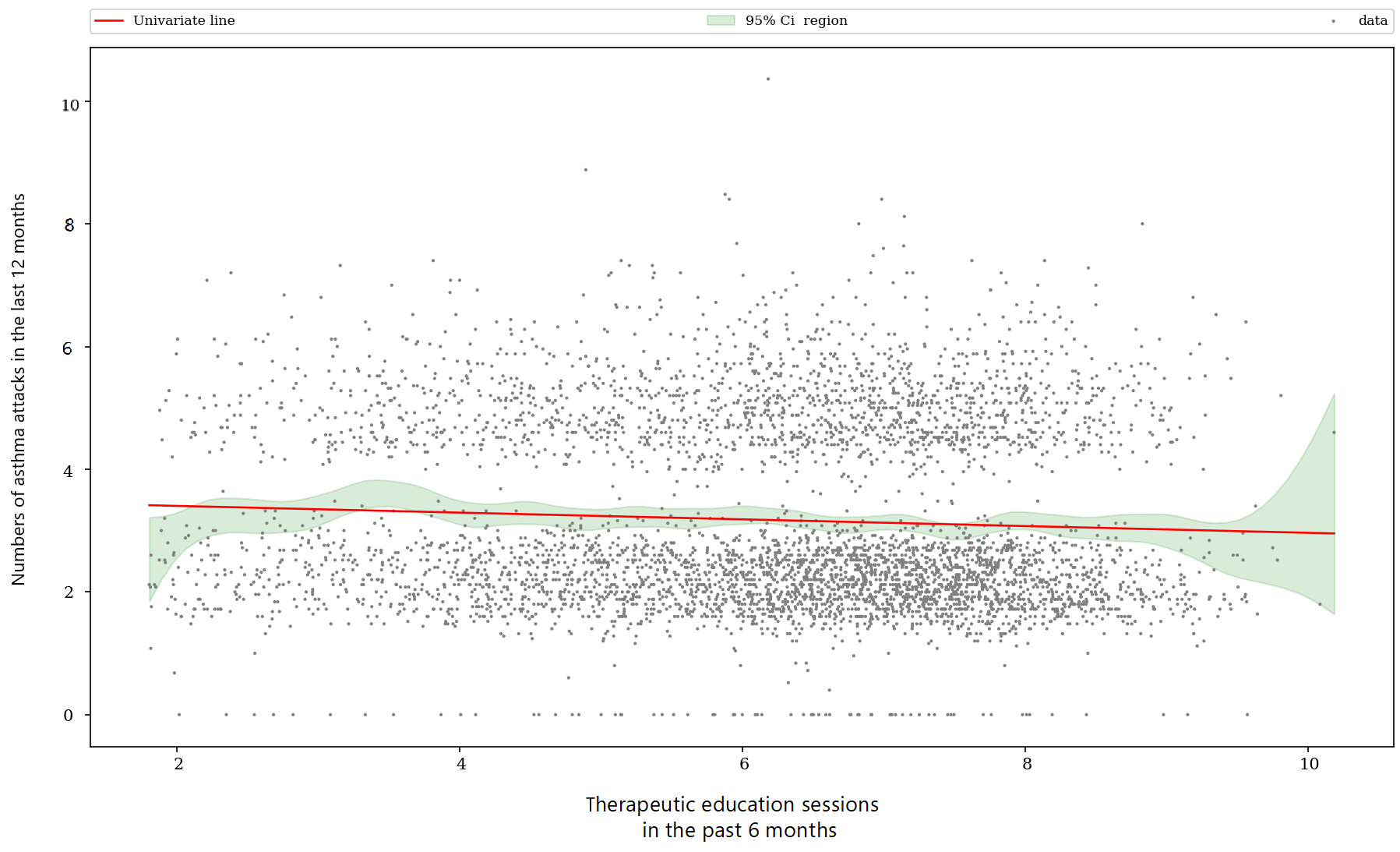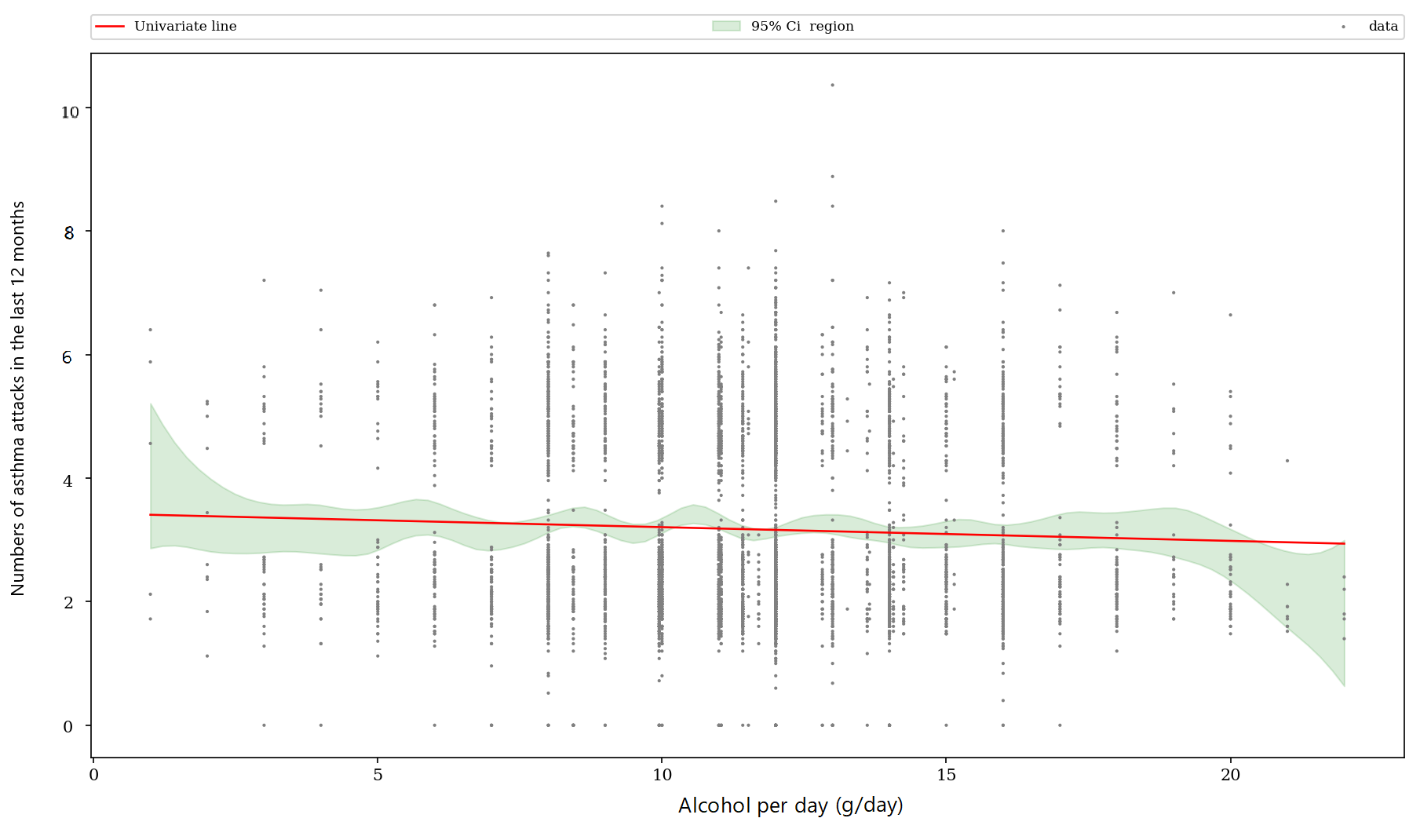NO, it is not within the green zone

The variables "Age", "Quality of life score" and "Number of rehabilitation sessions" are clearly non linear as we can see that the red line is quasi never within the green zone.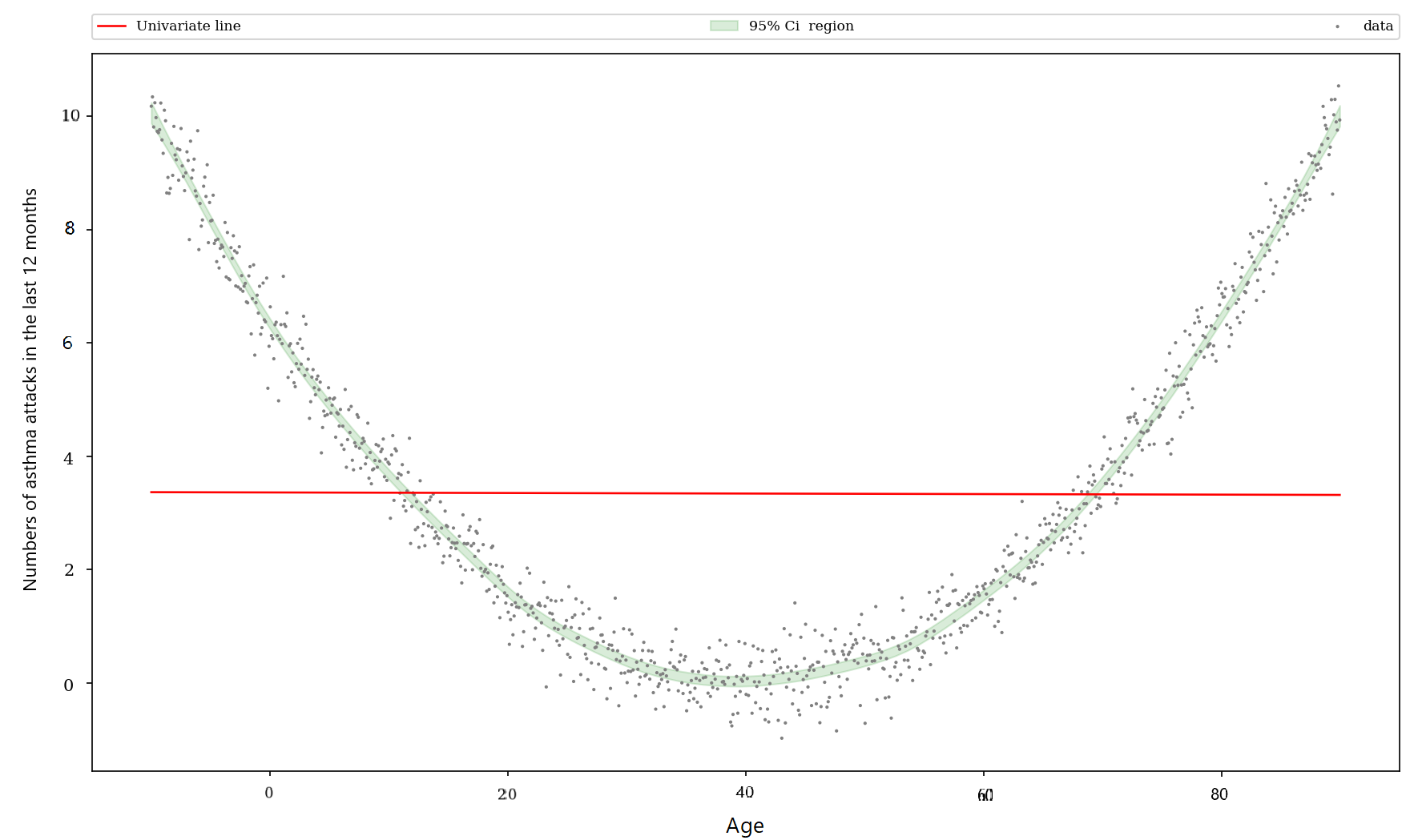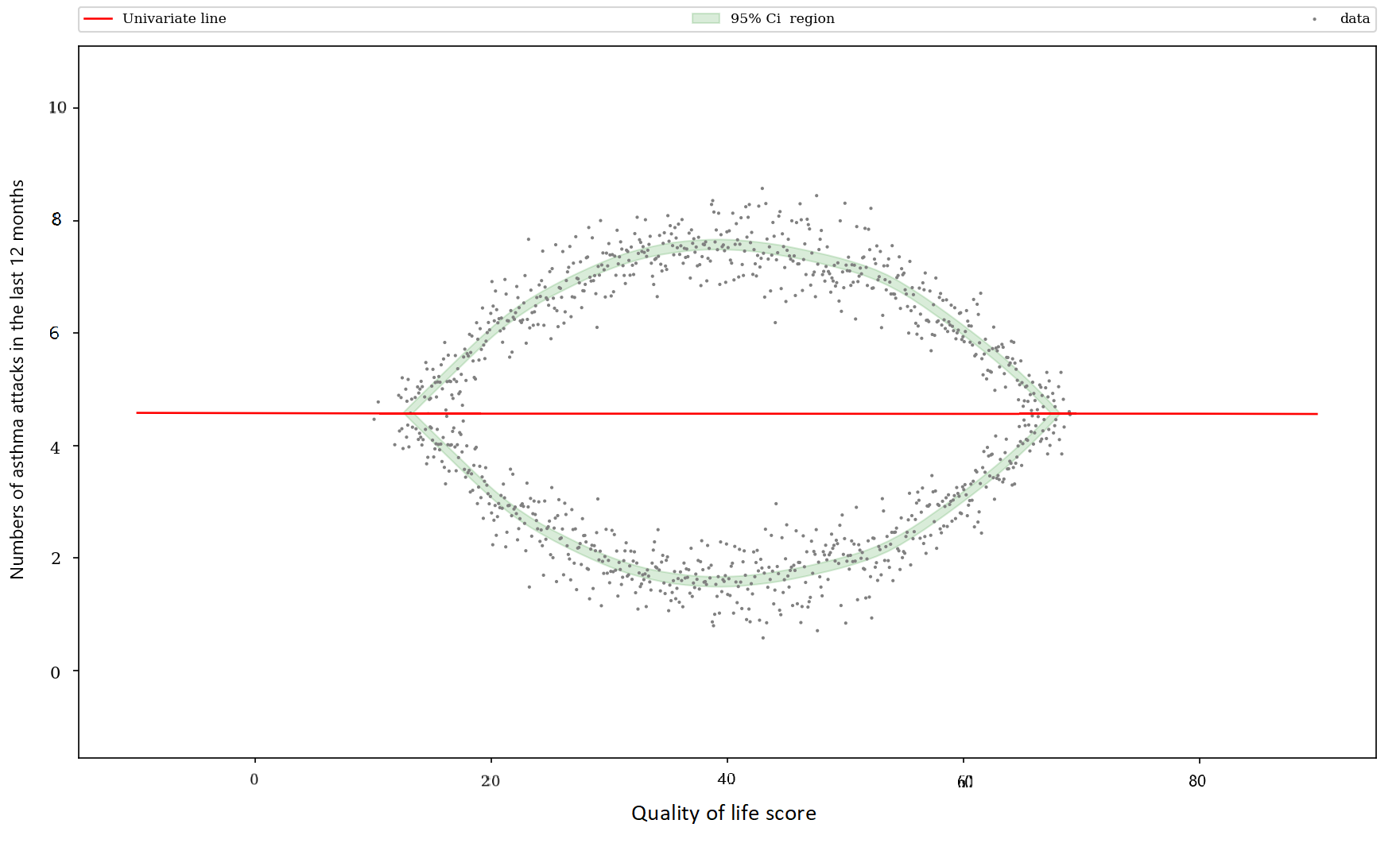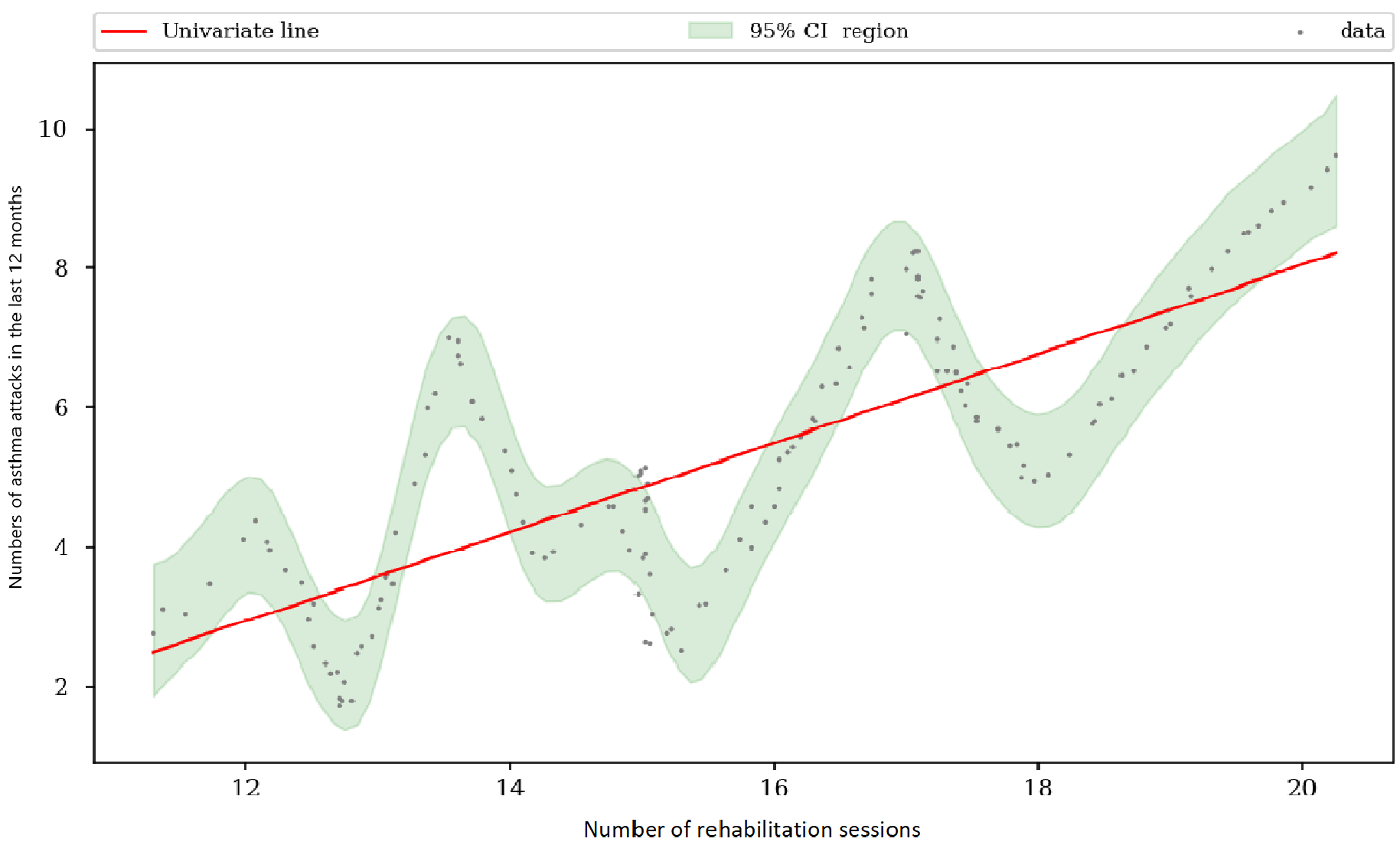YES, it is within the green zone BUT maybe non-linear ...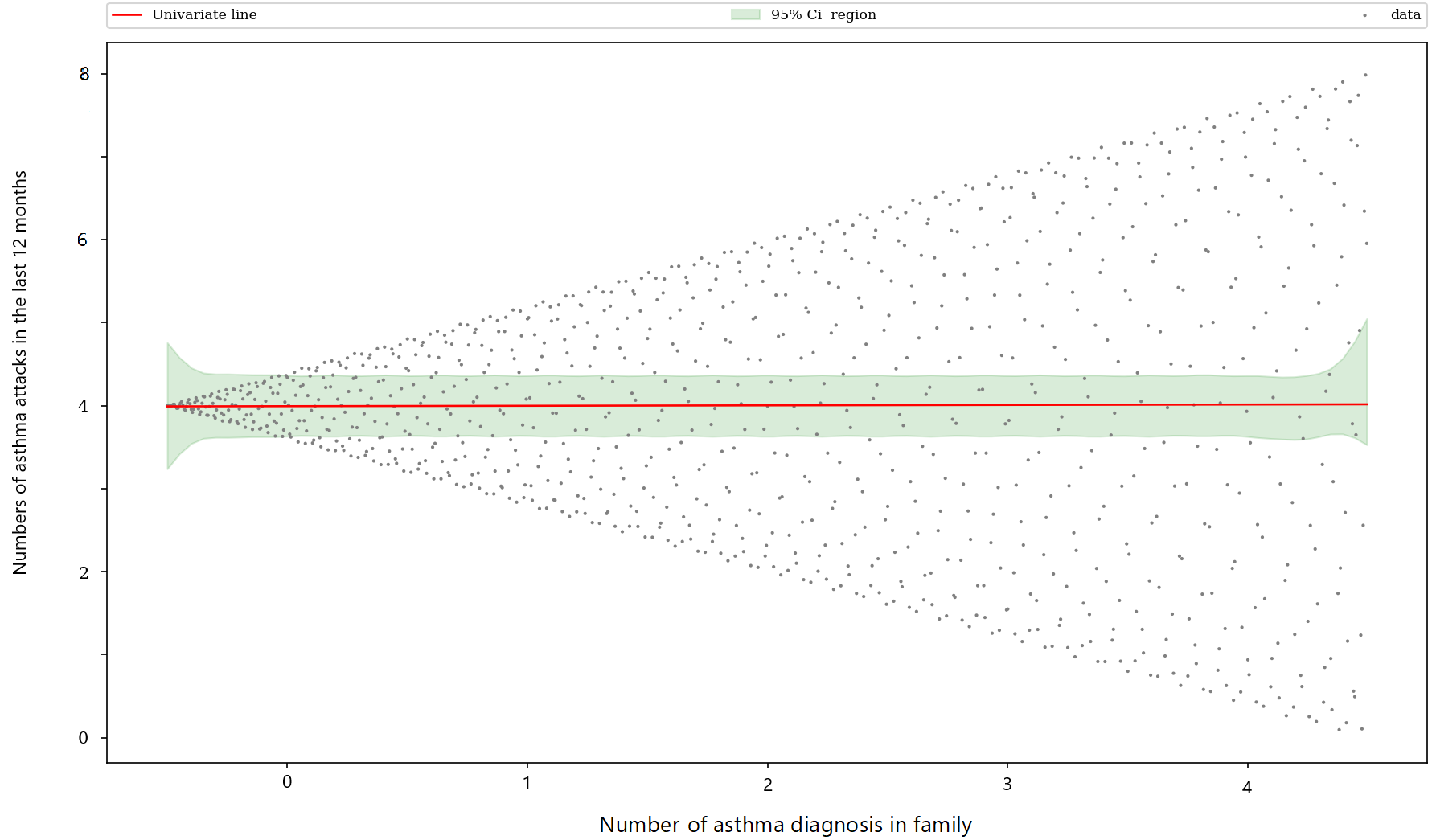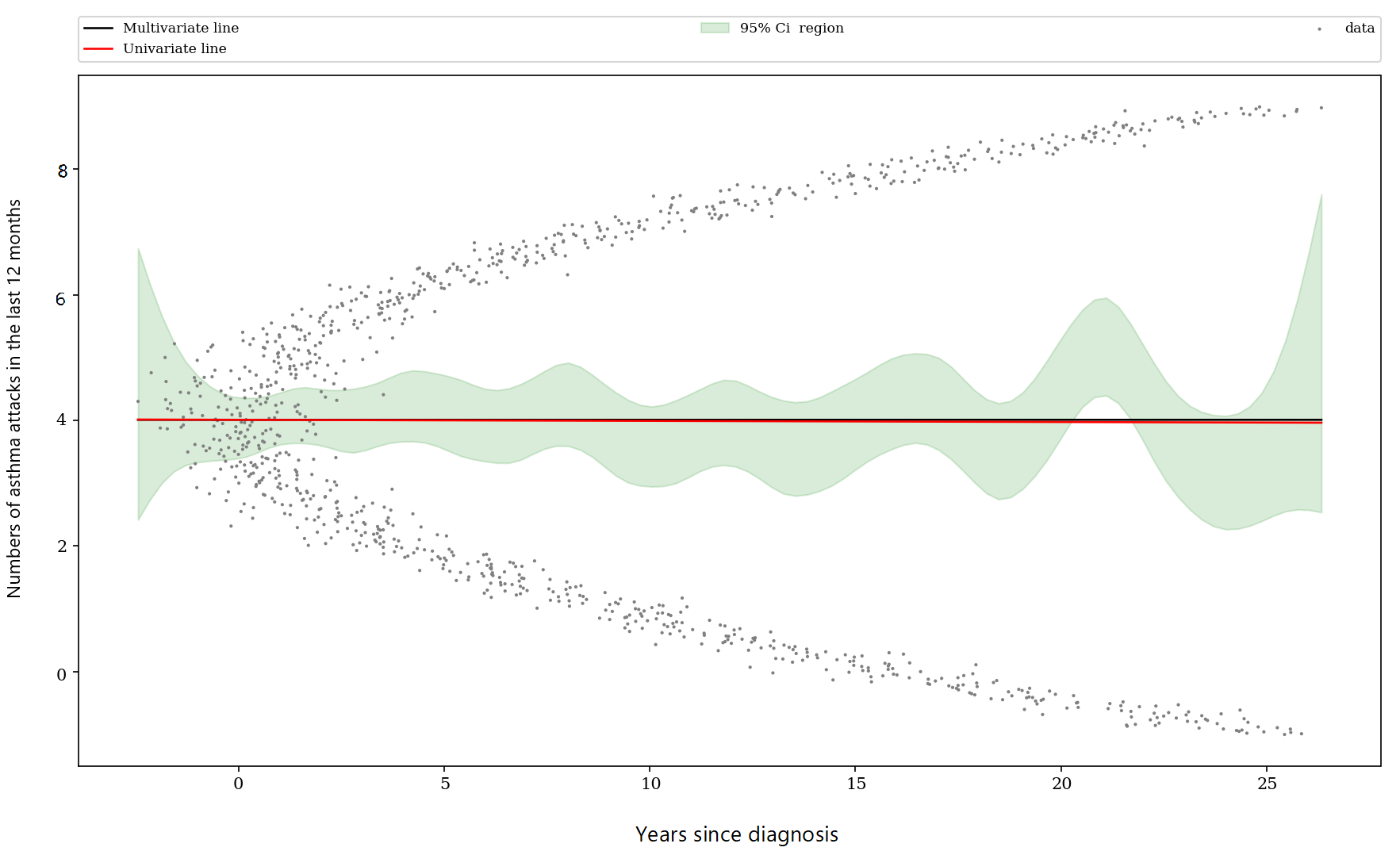For the variables "Years since diagnosis" and "Number of asthma diagnosis in family", the red line is generally within the green margin, the user can consider them as linear variables. But, we can discuss how there are specific cases that could be treated as non linear variables*.

* For the more experienced researchers

There are two special cases : "Years since diagnosis" and "Number of asthma diagnosis in family" are not linear, even if the red line is generally within the green zone. In fact, the point cloud shows that another mathematical relation could better explain the relationship between the variable to explain and the explanatory variable, as a curvilinear relationship for "Years since diagnosis" or polygonal or splines relationship for "Number of asthma diagnosis in family".

## Logistic

Same principle, the graphics are very similar, the only difference is that there is no scatter plot on them.

YES, it is clearly within the green zone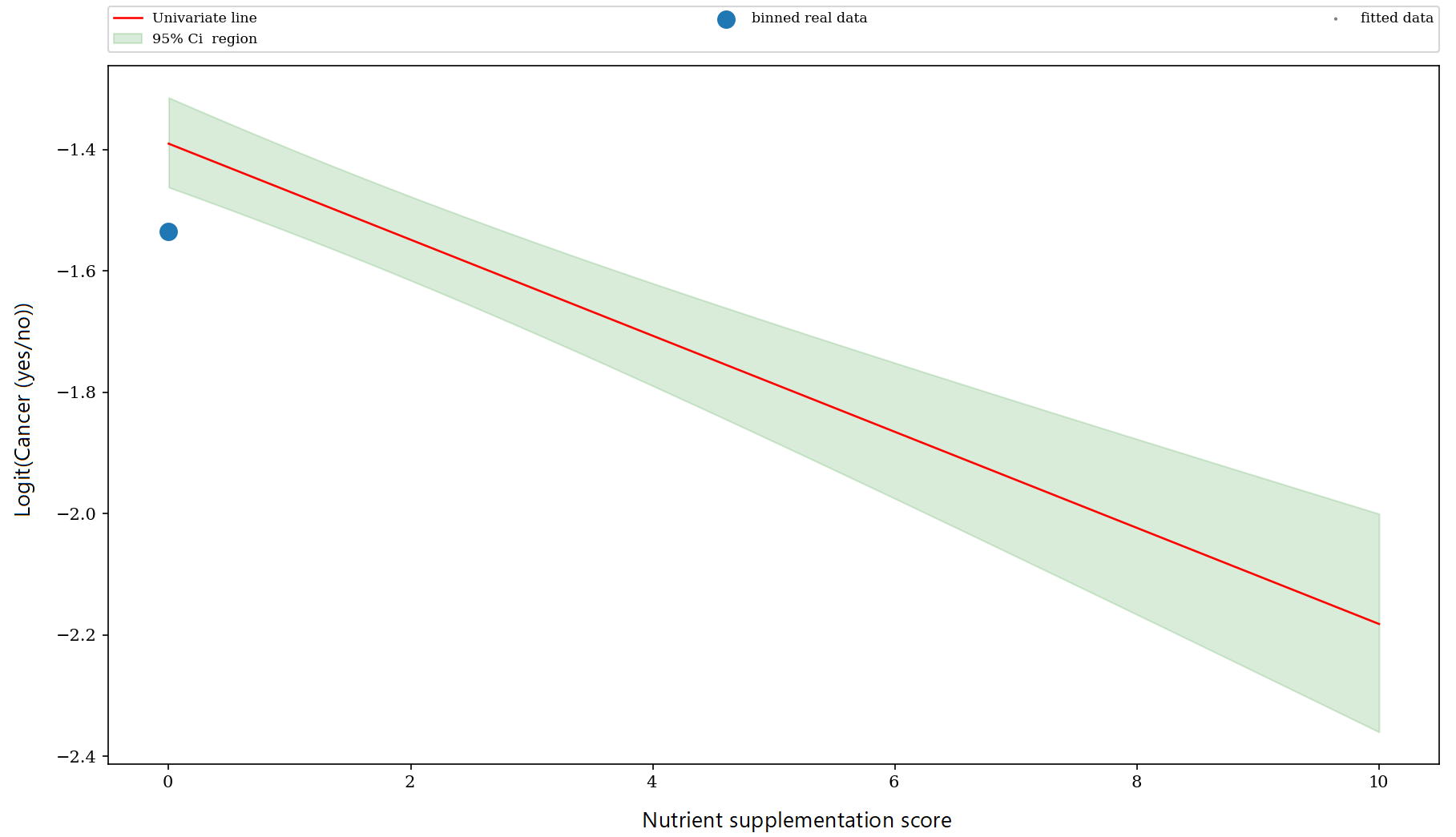NO, it is not within the green zone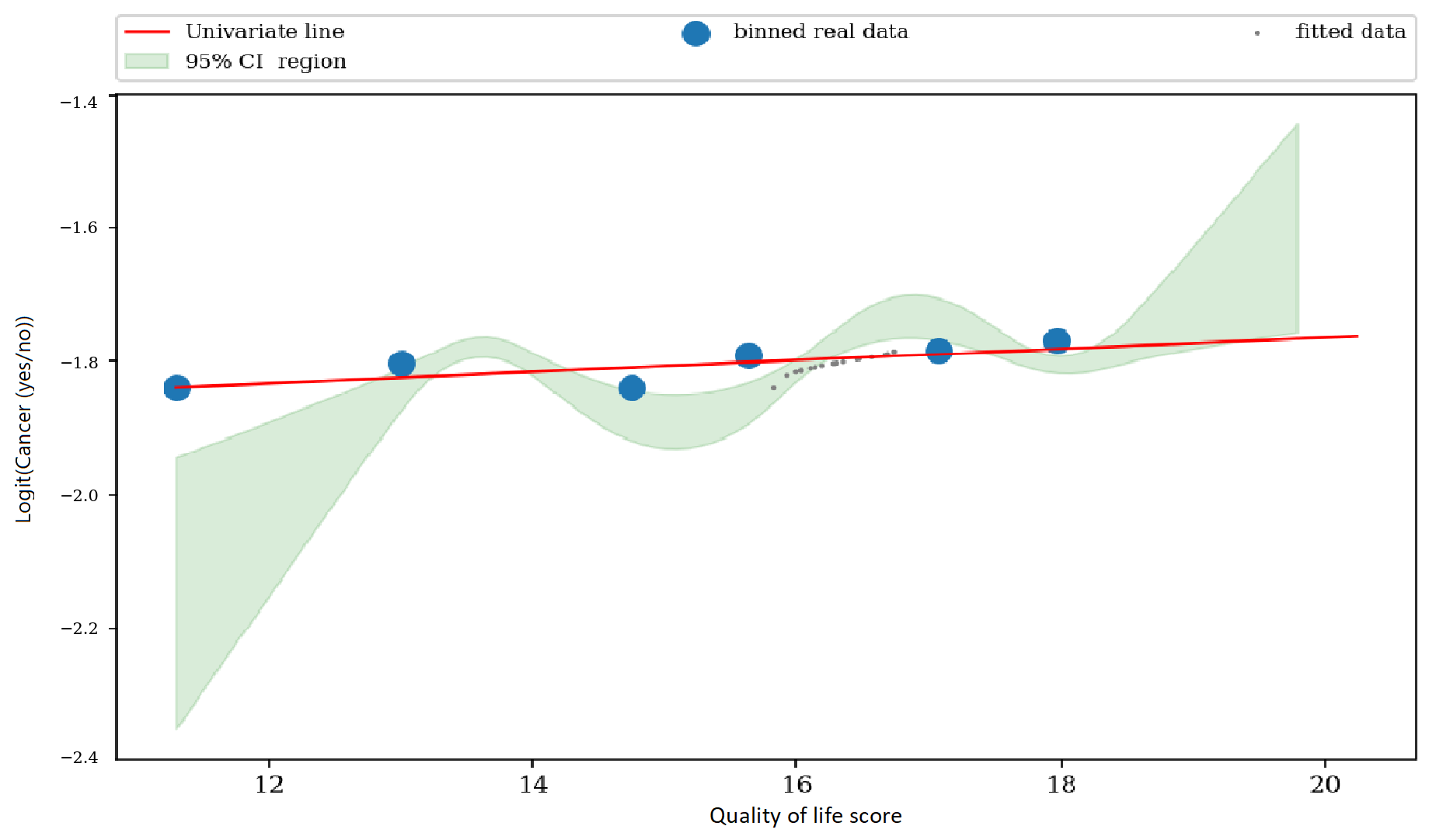For more details, see this book:

Garet J., Witten D., Hastie T. and Tibshirani R. An introduction to statistical learning with applications in R. Springer. 2013.
https://doi.org/10.1007/978-1-4614-7138-7